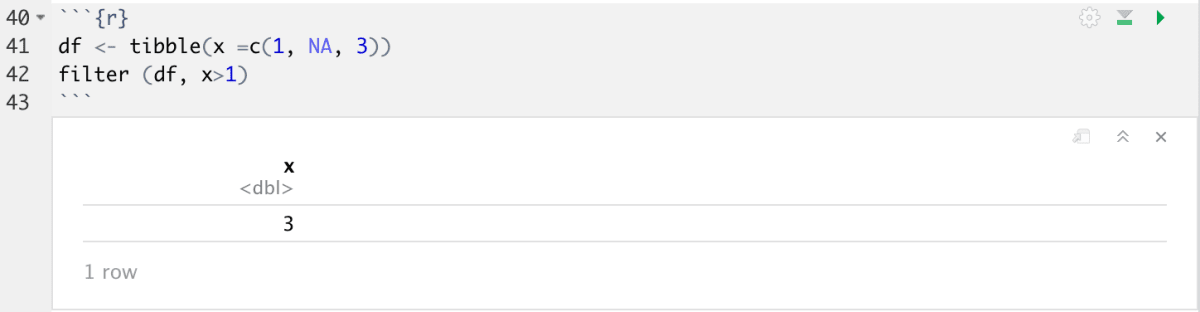Skip to content

# Understanding Data-frames Further

## Subsets, slicing and accessing data-frames

Subsetting is a useful process to extract specific information from a larger dataset.

### One Dimensional Object:

The syntax of subsetting a one dimensional object (like a vector or factor):

Example: I am going to create a vector (RB) which is a list, then subset some values from the list based on their indices.

```RB <- c("Red","Orange","Yellow","Green","Blue","Purple")
RB[c(1,3)]
```• `RB[c(1,3)]`returns the first and third elements in the list. To access elements in a data structure, the name of the data structure followed by a pair of square brackets `[]` is used. To further specify the elements to be extracted, their numerical indices are specified within the parentheses`()`.

### Two Dimensional Object:

The syntax of subsetting a two dimensional object (like matrices or data frames):

Example: Let’s use the data frame we explores in a previous section, `birthwt`

```bw_sum\$smoke
bw_sum\$birthweight[1:3]```• `bw_sum\$smoke` : the `\$` is used to subset the smoke column from our bw_sum data frame. The contents of the column are printed as a list.
• `bw_sum\$birthweight[1:3]` Again, a `\$` is used to subset the information in the birthweight column of the data frame. To selectively return the first 3 rows, I specify the argument, `[1:3]`

## Missing values

Missing values, or `NA`s (“not availables”) can make comparison quite tricky. `NA` represents an unknown value. Missing values are “contagious”: almost any operation involving an unknown value will also be unknown. Take a look at these examples. So confusing!Anything that has an `NA`, will also be `NA`.

Luckily, there is a solution! You can remove all NA values from your data.

The `filter()` function only includes rows where the condition is `TRUE`; it excludes both `FALSE` and `NA` values. If you want to preserve missing values, ask for them explicitly:

Example: we are creating a tibble (a simplified version of a data frame) containing the values 1, NA, and 3. Using the filter function, let’s find all values that are greater than 1.

```df <- tibble(x =c(1, NA, 3))
filter (df, x>1)```The result is a single value, 3. As the function doesn’t know whether NA is >1 or not, it excludes it for good measure!
If you want to account for missing values along with true statements, you can specify an additional condition `is.na(x)`

`filter(df, is.na(x) | x>1)`Removing NAs explicitly: You may see the `na.rm` argument used in some functions. This argument removes any NA values from the table.